By Topic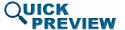• Abstract
• Authors
• Figures
• Multimedia
• References
• Cited By
• Keywords

# Calculation of the radar ambiguity function from time-domain measurement data for real-time, amplifier-in-the-loop waveform optimization

The ambiguity function is a measure of a radar's range and Doppler detection capability. Cognitive radar systems require the capability to adjust the waveform in realtime to obtain desired range/Doppler detection capability while meeting stringent spectral requirements. While the ambiguity function of the waveform input to the transmitter can be simulated, it is the ambiguity function of the transmitter power amplifier's output waveform that will be used for the detection. As such, it is very helpful to be able to measure the ambiguity function output from a power amplifier in the optimization process. This paper describes a technique that can be used to quickly calculate the ambiguity function for the output waveform from the radar amplifier as measured on an oscilloscope. Brief examination is also given to the effect of amplifier nonlinearity on the ambiguity function.

SECTION I

## INTRODUCTION

We are performing the ambiguity function measurements as part of a real-time waveform optimization based on obtaining a desired ambiguity function while meeting spectral mask constraints. The optimization uses a minimax approach described in a previous paper .

The literature shows evidence of working toward a realtime waveform optimization to maximize detection and meet spectral constraints. In the area of waveform optimization, the design of spectrally confined waveforms through variable-modulus techniques is described in , and then through constant-modulus techniques such as continuous-phase modulation in – and piecewise linear chirp optimization in . Skolnik discusses the connection of the ambiguity function with the waveform, including properties of frequency-modulated bursts, or chirps . Patton demonstrates optimization of the linear frequency-modulated (LFM) chirp design by tuning the nonlinear Fourier Series perturbations to the phase in . Holtzman and Thorp use the ambiguity surface as a weighted error criterion for waveform optimization in .  by Wong and Chung examines use of genetic algorithms to minimize the ambiguity function volume in different regions of the range-Doppler plane. In , Sussman applies least-squares optimization to the radar waveform problem. Blunt et al. and Cook demonstrate the use of continuous-phase modulation to minimize the spectral spreading of waveforms –. Finally,  and  represent our state of the art in optimizing radar systems. Measuring the ambiguity function of the amplifier's output in order to optimize the transmitted waveform is what motivates the work presented in this paper.

SECTION II

The radar ambiguity function is given by the following formula, which is described in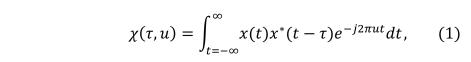TeX Source where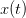$x(t)$ is the transmitted signal,$\tau$ is the difference in time from the actual time delay associated with the target, and$u$ is the frequency difference in Hertz from the actual Doppler frequency shift based on the target's true velocity. The ambiguity function shows the result from a range-Doppler correlation comparing offsets in time and Doppler shift from the actual time and Doppler shifts in a received signal. The ideal ambiguity function would show a high magnitude output at the center of the graph, which represents the case where$\tau$ equals the actual time shift of the received signal and$u$ equals the actual Doppler shift of the received signal. Any nonzero values of the ambiguity function anywhere other than the center of the graph represent potential range and Doppler shifts that could be mistaken for the correct range and Doppler shift.

The radar waveforms under consideration by our optimizations are typically linear FM chirps, which result in an ambiguity function that appears as a triangle in the ambiguity plane. Skolnik describes that the tilt angle of that triangle is determined by the ratio of the bandwidth of the chirp to the time duration required to sweep that bandwidth . Fig. 1 shows the magnitude plot on a linear scale for an ambiguity function of a chirp simulated in MATLAB. More examples for results from an ambiguity function can be found in .

Fig. 1. Ambiguity function magnitude for a linear FM chirp
SECTION III

## CALCULATING AN AMBIGUITY FUNCTION FROM MEASUREMENT DATA

As mentioned in the Introduction, the ambiguity function can be a useful tool for selecting the best waveform to use in a radar system. Our approach is to measure the waveform in the time domain with a 5 GHz oscilloscope, and then convert the waveform to baseband. In our approach, the waveform is down-converted to baseband to perform the real-time optimization, as in . The downconversion is necessary for speedy analysis and optimization due to the number of samples required to measure the full output signal from the amplifier at our carrier frequency of 3.3 GHz. Sampling at exactly the Nyquist rate, a 10 microsecond chirp at a carrier frequency of 3.3 GHz requires over 66,000 samples to represent. The resulting ambiguity function from those samples is a square matrix 66,000 by 66,000, so a minimum of 4.4 billion matrix elements would need to be calculated in that ambiguity function, far more than can be reasonably calculated in a real-time optimization. It is desirable to calculate the ambiguity function for the output waveform from the amplifier, however, because the amplifier's distortion will have an impact on the resulting ambiguity function.

In calculating the ambiguity function for measured data, we down-convert and down-sample the measured data. The technique required to do that is a fairly well known technique, which works as shown in Fig. 2. In the down-conversion process, the signal is separated into its in-phase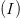$(I)$ and quadrature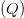$(Q)$ components. The signal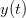$y(t)$ in Fig. 2 represents the signal measured at the output of the amplifier. That signal is then mixed with a sine and a cosine at the carrier frequency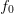$f_{0}$. That signal is then passed through a low-pass filter to remove the high-frequency duplicates from the mixers. To maintain the same number of samples per cycle of the highest frequency component, the signal is down-sampled by the ratio of the carrier frequency to the bandwidth of the baseband chirp being used. For the measurements shown at the end of this paper, that ratio is 3.3 GHz to 10 MHz, which means that only one of every 330 samples is required to represent the chirp signal at baseband. The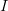$I$ and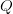$Q$ data represent the in-phase and quadrature components of the baseband signal and can finally be recombined in order to calculate a much smaller ambiguity function.

Fig. 2. Process for Down Converting and Down Sampling

We used measurements in our nonlinear test bench to measure the ambiguity function of chirp waveforms . The measurement setup consists of the following: an Agilent N5182A signal generator, a Skyworks SKY65017 InGaP amplifier as the device under test, an Agilent E3647A DC power supply, a Maury Microwave Automated Tuner System (ATS) load-pull setup, an Agilent N1911A power meter, an Agilent E4407B spectrum analyzer, and a LeCroy Wavemaster 8500 oscilloscope. The signal generator is used to convert the programmed chirps to the carrier frequency of 3.3 GHz used in these experiments, and the Maury load-pull system is a key component of the circuit portion of the optimization that is described in . The DC power supply provides a 9 V bias to the Skyworks amplifier. The oscilloscope is used for the actual measurements of the time domain chirp signal.

Fig. 3 shows the magnitude plot for a measured ambiguity function using the measurement system described in the previous paragraph without the amplifier in the loop. This provides a reference to confirm that the process described in the previous paragraph will provide accurate results for the ambiguity function. This final check allows confident analysis of the effects of the amplifier's distortion on the ambiguity function. While the axes in Fig. 3 are labeled as delay$\tau$ and Doppler shift$u$, they are given in points rather than in Hz or seconds. These points represent the entries involved in the ambiguity function matrix calculation, and can be converted to seconds (for delay) and Hertz (for Doppler frequency. The measured chirp used to calculate this ambiguity function is a down-chirp with 5 MHz bandwidth.

Fig. 3. Ambiguity function magnitude for a measured chirp

In analyzing the effects of the amplifier's distortion on the ambiguity function is to convert the ambiguity function to a logarithmic scale instead of the linear scale shown in the previous figures. Converting to a logarithmic scale allows for easier comparison of the distorting effects on the various chirps. Fig. 4 shows the default view of a logarithmic magnitude (dB) plot of an ambiguity function for an up chirp with 10 MHz bandwidth without the amplifier in the measurement. Fig. 5 shows the result for the same chirp, only with the amplifier in the measurement, with the amplifier terminated in the load impedance providing maximum power-added efficiency (PAE), as determined by a separate load-pull measurement. Fig. 6 shows the result for the same chirp and amplifier, but for this test the amplifier is terminated in the load impedance providing the minimum adjacent-channel power ratio. These figures still do not make the distorting effects obvious, however, so the figures are then rotated to look along the edge of the primary peak of the ambiguity function.

It is insightful to examine rotated plots of the ambiguity function; that is, to view the main ambiguity ridge “head-on.” Fig. 7 shows the head-on view of the ambiguity function of the waveform measured without the amplifier in the loop. Fig. 8 shows the rotated plot of the ambiguity function of the amplifier's output waveform, where the amplifier is terminated in the maximum-PAE load impedance, and Fig. 9 shows the rotated plots of the ambiguity function of the amplifier's output waveform when terminated in the minimum-ACPR load impedance.

Several effects can be notice through examination of the head-on view of these measurements. Unlike Fig. 7, which examines the amplifier's input waveform, Fig. 8 reveals that a “spreading” of the ambiguity occurs as a result of the amplifier's nonlinearity. In Fig. 7, the difference between the peak of the main ambiguity ridge and the maximum ambiguity in the adjacent side-region is approximately 45 dB. However, in Fig. 8, with the amplifier in place, this difference is reduced to approximately 34 dB. This effect of distortion is significant: the interpretation of these results is that the distortion of the amplifier impacts the range/Doppler precision of detection in some regions parallel to the main ambiguity ridge. Fig. 9 shows that when the amplifier is terminated in the load impedance resulting in minimum ACPR, the signal energy decreases due to decreased efficiency, and thus the height of the main ridge decreases. Further studies will examine the significance of the “ambiguity spreading” and make a theoretical connection to amplifier nonlinearities.

Fig. 4. Logarithmic ambiguity function magnitude for a measured chirp with no amplifier
Fig. 5. Logarithmic ambiguity function magnitude for a measured chirp with an amplifier, where the load impedance is chosen as the impedance providing the maximum power-added efficiency (PAE)
Fig. 6. Logarithmic ambiguity function magnitude for a measured chirp with an amplifier, where the load impedance is chosen as the impedance providing the minimum adjacent-channel power ratio (ACPR)
Fig. 7. Logarithmic edge view ambiguity function magnitude for a measured chirp with no amplifier
Fig. 8. Logarithmic edge view ambiguity function magnitude for measured chirp with an amplifier, where the load impedance is chosen as the impedance providing the maximum power-added efficiency (PAE)
Fig. 9. Logarithmic edge view ambiguity function magnitude for measured chirp with an amplifier, where the load impedance is chosen as the impedance providing the minimum adjacent-channel power ratio (ACPR)
SECTION IV

## CONCLUSIONS

This paper has described a technique which allows the radar ambiguity function to be quickly calculated from a time-domain measurement of a radar chirp waveform. The measurement demonstrated in this paper is critical to the realtime waveform optimization of a cognitive radar transmitter with an amplifier in the loop. This allows for the effect of amplifier distortion to be included in the measurement of the output waveform actually used for detection, and will result in more accurate optimizations and the ability to generate desired radar waveforms more precisely. Examination of the ambiguity functions output from a test amplifier show a significant effect of amplifier distortion on the ambiguity function, confirming the need for the measurements described in this work. This paper provides a basis for measurements to aid in real-time optimization, and sets the foundation for further studies into the effects of amplifier nonlinearity on the radar ambiguity function. In future work we expect to use this new measurement to implement measurement-based waveform optimization for radar transmitters.

### Acknowledgments

This work has been funded in part by the National Science Foundation (Award No. ECCS-1343316) and the United States Naval Research Laboratory. The authors wish to thank David Moon, formerly of Baylor University, for his work and insight on ambiguity functions that has benefitted the analysis presented in this paper.

## Footnotes

No Data Available

## Corrections

None

This paper appears in:
Microwave Measurement Conference, 2013 82nd ARFTG
Conference Date(s):
18-21 Nov. 2013
Conference Location:
Columbus, OH
On page(s):
1 - 5
E-ISBN:
No Data Available
Print ISBN:
No Data Available
INSPEC Accession Number:
14112539
Digital Object Identifier:
10.1109/ARFTG-2.2013.6737360